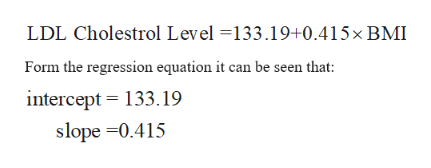# A regression of the LDL cholesterol level on the Body Mass Index (BMI) is shown below:LDL Cholesterol Level = 133.19 + 0.4151*BMI. Which of the following is FALSE?  It is estimated that for every additional unit of BMI, the Level of LDL Cholesterol increases by about 0.4.  It is estimated that for the person with zero BMI, the LDL Cholesterol Level is about 133.  If one person has BMI of 20, then it is estimated that the LDL Cholesterol Level is about 141.  If the LDL Cholesterol Level of one person is 145, then this equation indicates that this person’s BMI is about 30.  One person’s LDL Cholesterol Level is 143 and BMI is 25. Its residual is about 5.

Question
2 views
1. A regression of the LDL cholesterol level on the Body Mass Index (BMI) is shown below:
LDL Cholesterol Level = 133.19 + 0.4151*BMI. Which of the following is FALSE?

 It is estimated that for every additional unit of BMI, the Level of LDL Cholesterol increases by about 0.4. It is estimated that for the person with zero BMI, the LDL Cholesterol Level is about 133. If one person has BMI of 20, then it is estimated that the LDL Cholesterol Level is about 141. If the LDL Cholesterol Level of one person is 145, then this equation indicates that this person’s BMI is about 30. One person’s LDL Cholesterol Level is 143 and BMI is 25. Its residual is about 5.
check_circle

Step 1

According to the provided information, regression line of the LDL ...help_outlineImage TranscriptioncloseLDL Cholestrol Level =133.19+0.415×BMI Form the regression equation it can be seen that: intercept = 133.19 slope =0.415 fullscreen

### Want to see the full answer?

See Solution

#### Want to see this answer and more?

Solutions are written by subject experts who are available 24/7. Questions are typically answered within 1 hour.*

See Solution
*Response times may vary by subject and question.
Tagged in

### Other